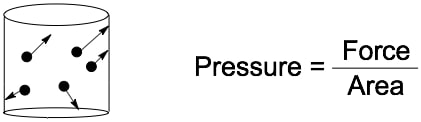## GOB Chemistry

Learn the toughest concepts covered in your GOB - General, Organic, and Biological Chemistry class with step-by-step video tutorials and practice problems.

Gases, Liquids and Solids

# Pressure Units

Pressure is the force that is exerted within a given volume of space.

Understanding Pressure
1
concept

## Pressure Units1m
Play a video:
the S. I. Unit for pressure is the pascal, abbreviated as P. A. And it's named over the french mathematician Blaise pascal when it comes to pressure. Let's think about what that really means. When it comes to gasses, they can be seen as a collection of molecules that move in random directions in straight lines. So here we have an image of a container inside of these containers. We have these blue dots which represent gas molecules. All they're doing is bouncing around randomly bumping into the sides of the container, bumping into each other. How does this apply to pressure? Well, pressure is the force that a gas exerts on the wall of its container connected to pressure. Are two ideas F which equals force in S. I. Units of newtons and A. Which represents area and it's S. I. Unit is in meters squared. So pressure itself is just the force that the gasses exert over an area. So here we're gonna say that pressure equals force over area. This idea of gasses existing within containers are gonna be delved deeper into. When we talk about ideal gasses and eventually non ideal gasses

Pressure represents the force that a gas molecule exerts against the walls of its container. It can be depicted as force per area.2
example

## Pressure Units Example 148s
Play a video:
in this example question. It says what happens to the pressure if the same amount of gas molecules is transferred from a five liter container toe a 10 liter container? Remember, pressure equals pressure equals force over area. They're saying it's the same amount of gas molecules. Since it's the same amount of gas molecules, they should exert the same type of force. But what's happening? We're going from a five liter container toe, a 10 liter container, so our area is going up the size inside the container is increasing, so if force is staying the same but areas increasing, that's gonna cause my pressure to decrease. So here, our best option would have to be Option B, where it's going to decrease.
3
concept

## Pressure Units1m
Play a video:
additional non S i units for pressure used by chemists are atmospheres, which is ATM millimeters of mercury, which is MM H G. And then just tour. Now these units for pressure have their own pressure value, which can be related to one another. Now, remember, if you see a purple box that people box means you have to memorize it because usually it's not given to you in terms of a quiz or exam. Now the pressure values associated with atmospheres Millimeters of Mercury and Tour are one 7 60 and then 60 again. Now the less commonly known ones. Pascal's cable, Pascal's bars and slides are in purple boxes, meaning they're not usually expected to be memorized. They'll be given to you in some way within the question or formula sheet. Now they're pressure. Values are 1.13 to 5 times 10 to the five kilo. Remember, Kilo was 10 to the three, so this is one on 1.3 to 5 bars. Here is 1.13 to 5. And then finally, if we look at Sai Sai is 14.696 now, these numbers relate to one another. We said that there a way to relate to each each other to each other. That means that one atmosphere equals 7 millimeters of mercury, or 1.13 to 5 bars equals 7, 14, 696 size. So these are just ways of them connecting toe one another. But again, it's the first three that are gonna be the most important. Even though they're non s I units, they're the ones most commonly used by chemist. So now that we know these different types of pressure values, let's continue onward to an example quest.
4
example

## Pressure Units Example 22m
Play a video: